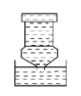$\text{A.}$ 仍是满鑵水 $\text{B.}$ 水将流出一部分 $\text{C.}$ 水将全部流出 $\text{D.}$ 以上说法都不对

A